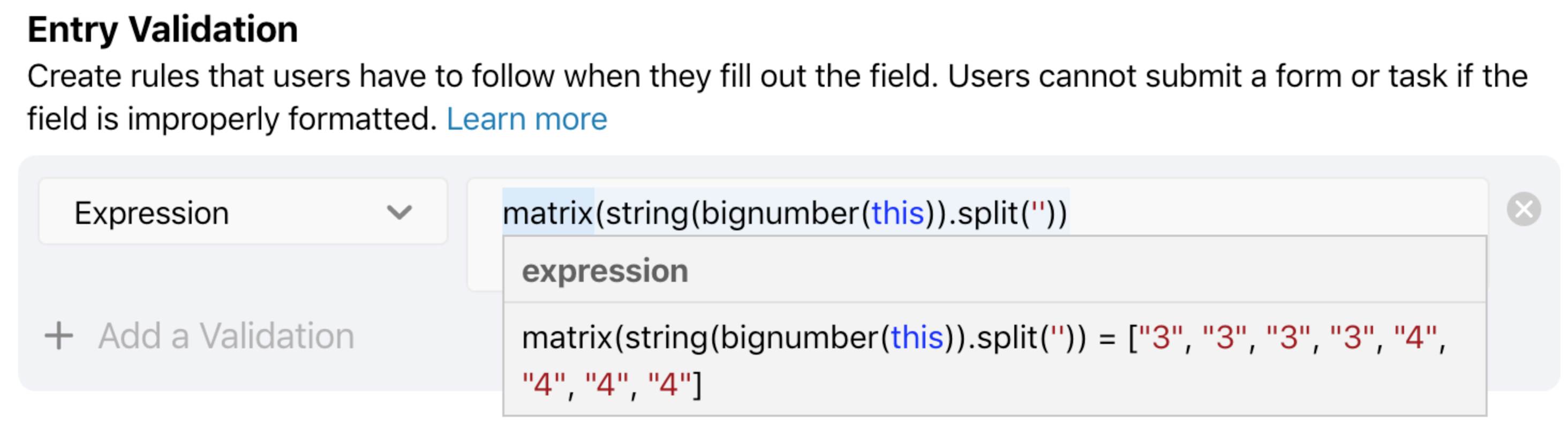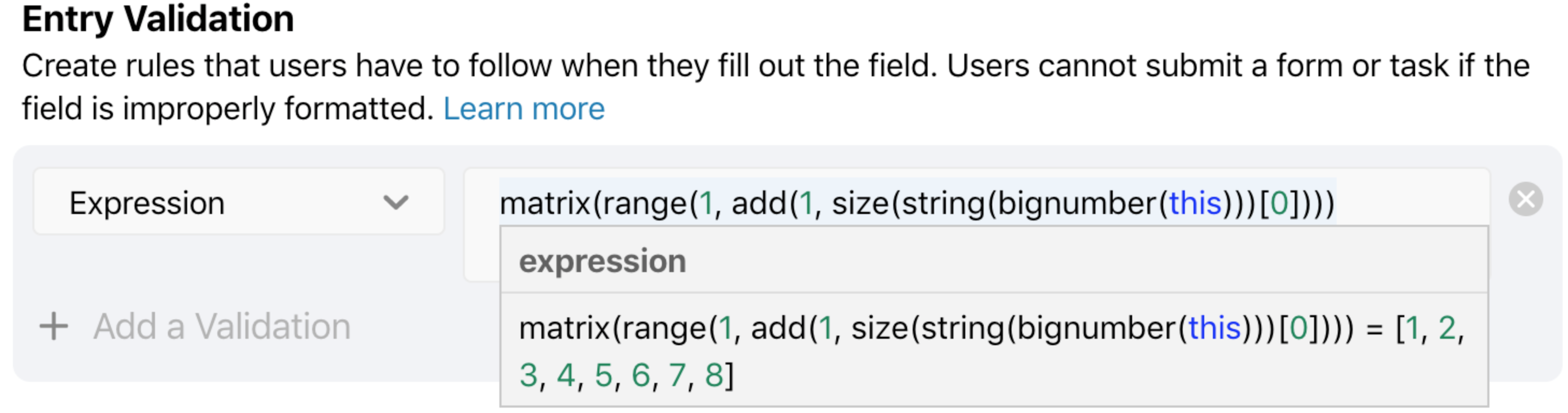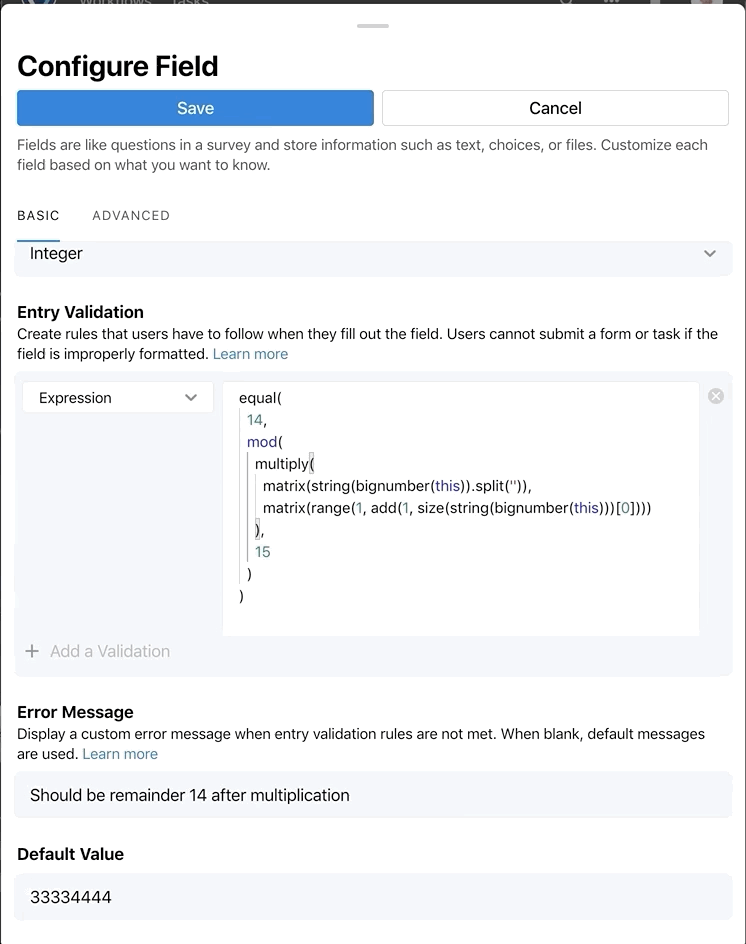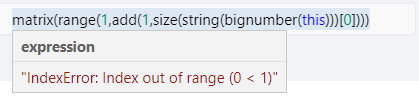# Data Validation with more complicated math

I would like to perform data validation on a webform entry based upon the length of the data entered. Something similar to this:
Number entered: 33334444
Calculation: mod(((1*3)+(2*3)+(3*3)+(4*3)+(5*4)+(6*4)+(7*4)+(8*4))/15) = 14
Result: True
I have looked at the data validation available in fields for webforms and in regex, but they don't appear to do this. Any suggestions?

Tagged:

• Hi @Cora_507115 You can use Expression Validation for your field to validate the entry and prevent the web form from being submitted if the result does not evaluate to true.

I tried out this simple expression using the `mod` formula:

```mod(this, 9) == 1
```

You use `this` to refer to the field value that is entered.

In the example above, the form would prevent me from submitting unless the number I entered had a remainder of 1 after being divided by 9.

I suggest you review the [Expression Validation help article] and then review the documentation and test out formulas at mathjs.org. Let us know if you could use any additional guidance as you set up your expression.

• Hi @Jeff_291769 , Thank you for the mod information. I'm thinking something like the formula below might work, but I believe I'd need to somehow pad the "this" to have leading zeros and Catalytic doesn't appear to like the "str.charAt()" - I have a lot to learn yet, but am not seeing string manipulation in the help. Can you provide any direction?

mod(str.charAt(0)*1+str.charAt(1)*2+str.charAt(2)*3+str.charAt(3)*4+str.charAt(4)*5+str.charAt(5)*6+str.charAt(6)*7+str.charAt(7)*8,15)==14

• edited August 19

@Cora_507115 You can refer to the first digit using `number(string(this))`, the second digit using `number(string(this))`, etc.

In that syntax, we're converting the value (`this`) to text using the `string` function and using the number in the square brackets to denote the specific character in the string. The `number` function at the beginning converts the string back into a number, so that you can use it in your formula.

• Let me add another twist... the number I'm validating may be of variable length when entered. In my example above, I showed multiplying the 8 digits by 1-8, but if there are 9 digits, they need to get multiplied by 1-9 or 6 digits, 1-6....

• @Cora_507115 Is it essential that this be validated on the form? Or could it be validated after the form is submitted?

• edited August 19

@Cora_507115 I updated my comment above to confirm the syntax for referencing the different digits. The reference to the first digit will be `number(string(this))`.

• It will make the process much less customer friendly to do afterward as nothing can proceed without a valid number. As I understand it, this would mean essentially creating an infinite loop of emails until a valid number is entered.

• Hi @Cora_507115, can you see if this Entry Validation expression satisfies your use case?

```equal(
14,
mod(
multiply(
matrix(string(bignumber(this)).split('')),
),
15
)
)
```
• @Tom_121527 I'm having some trouble, but I may be doing inserting it incorrectly.... I believe I replace "this" with the field name and remove the formatting (spaces and LF) so it is all one line. Is that incorrect or is there anything else that would be needed to insert this as an expression validation?

• @Tom_121527 It's hanging up on the split. Not splitting. So the first matrix is still just "this" rather than all of the digits in "this".

• Hi @Cora_507115, you do need `this`. It's a special keyword that will evaluate to whatever the value of the field happens to be, for example integer `33334444`. The formatting is totally optional. The expression should work with or without the spaces and new lines.

If you paste the above expression in as is, you should see it work against the Default Value of your field, assuming I'm correctly understanding the math you're looking to perform.

For reference,

## Matrix 1 (when default value is integer `33334444`):## Matrix 2 (when default value is integer `33334444`):## Validation:• @Tom_121527 Could there be some kind of setup difference between my Catalytic and yours? Matrix 2 gives me• Hi @Cora_507115, I will be in contact via our [email protected] email address to help further this Community discussion.